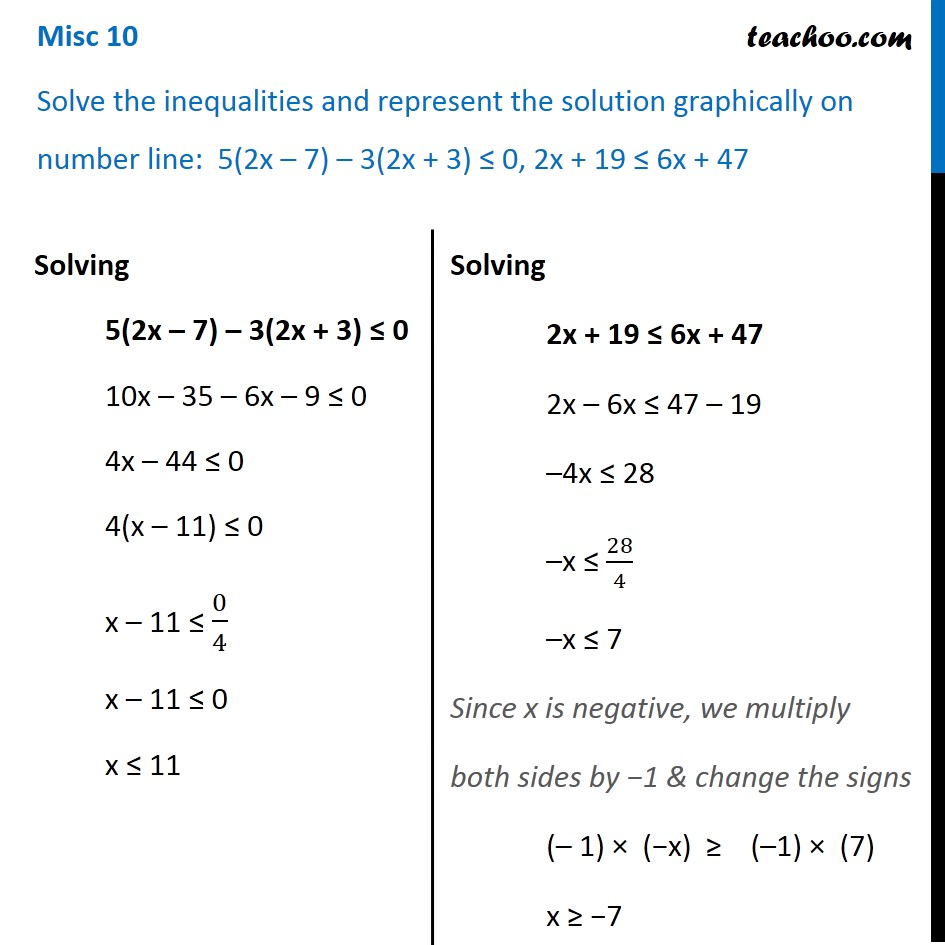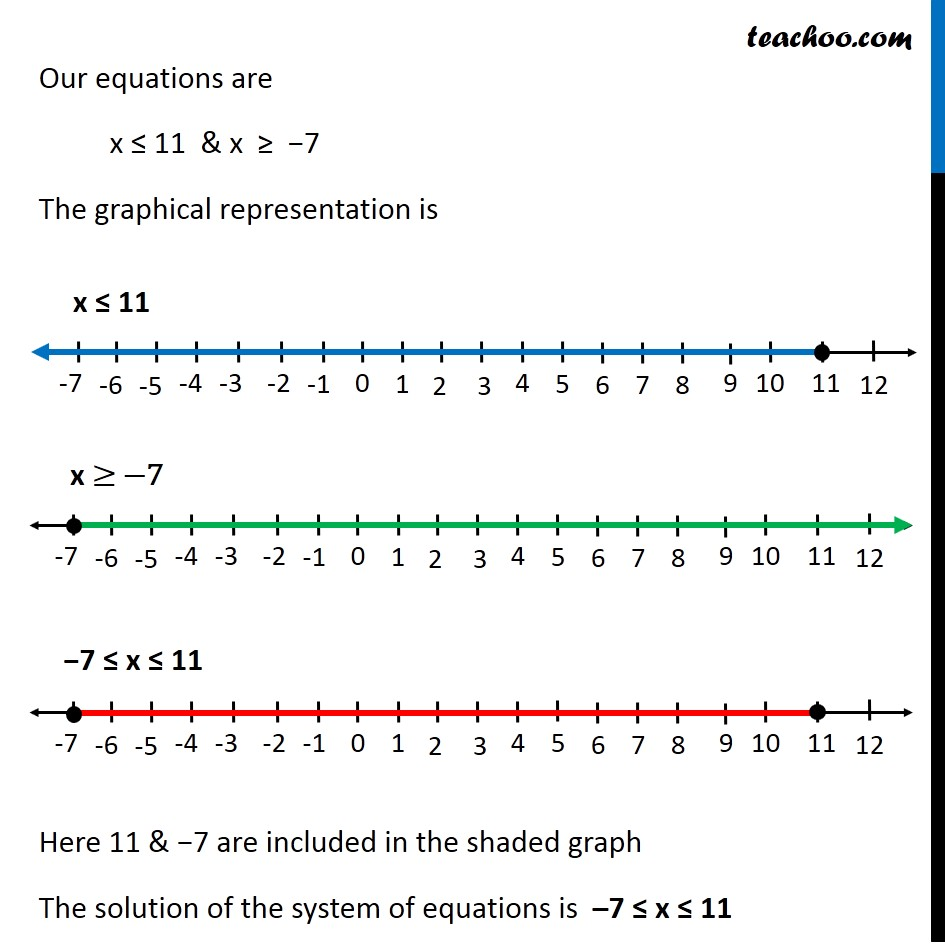Miscellaneous

Chapter 6 Class 11 Linear Inequalities
Serial order wiseMaths Crash Course - Live lectures + all videos + Real time Doubt solving!

### Transcript

Misc 10 Solve the inequalities and represent the solution graphically on number line: 5(2x – 7) – 3(2x + 3) ≤ 0, 2x + 19 ≤ 6x + 47 Solving 5(2x – 7) – 3(2x + 3) ≤ 0 10x – 35 – 6x – 9 ≤ 0 4x – 44 ≤ 0 4(x – 11) ≤ 0 x – 11 ≤ 0/4 x – 11 ≤ 0 x ≤ 11 Solving 2x + 19 ≤ 6x + 47 2x – 6x ≤ 47 – 19 –4x ≤ 28 –x ≤ 28/4 –x ≤ 7 Since x is negative, we multiply both sides by −1 & change the signs (– 1) × (−x) ≥ (–1) × (7) x ≥ −7 Our equations are x ≤ 11 & x ≥ −7 The graphical representation is Here 11 & −7 are included in the shaded graph The solution of the system of equations is –7 ≤ x ≤ 11